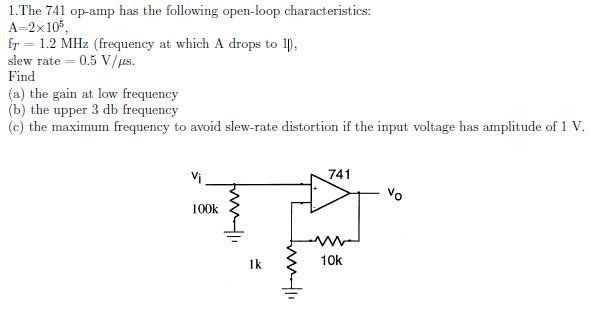# Upper 3db Frequency of Op Amp Help

• mmmboh

#### mmmbohSo I did the first part, and got the gain as 11.
I also did part c, and got approximately 80khz,
but I'm not sure what to do for b).
I know that at the 3db frequency, the voltage is at 1/20.5 of it's amplitude.
So can I just use the equation f=(slew rate)/(2pi*A), and set A to 1V/20.5, and solve for f? In which case f=112.5khz
If not, what should I do?

Thanks!

Questions:

(b) What does "upper 3 dB frequency" mean? Does it mean the frequency at which the gain rolls off 3 dB from the value of 11 or does it mean the frequency at which the gain is 3 dB. (Does "upper" refer to gain or frequency in the question?)

(c) Does the input voltage amplitude of 1 V represent a peak to peak, peak or RMS measurement?

The amplitude is 1 V. And I believe for b) it's when the gain is 3db, although your guess is as good as mine. This is due tomorrow so I don't have time to ask the teacher.

I get a different answer for (c) than you do. Here's how I thought about it.
What is the slope at 180 deg? (or the absolute value of the slope) That value must relate to the slew rate of the opamp of 0.5 V/uS. How many microseconds long must one cycle be so that the slope of the sine wave when it crosses the x-axis is equal to 0.5 V/uS?

If you show me your work I'll make some suggestions.

Well I said f=(slew rate)/(2pi*A) = (0.5/us)/2pi=80khz. Why doesn't think work?
Any thoughts on b?

Thanks.

My mistake, you are correct about the slope.

(b) The voltage gain drops by 2 dB per 1 dB change in frequency. An output gain of 3 dB should be at a frequency 1.5 dB less than 1.2 MHz. Can you figure that out?

Hm...1.5=10*log(1.2MHz/f), f=0.85 MHz?...Or should the f be in the numerator of the log since its upper 3db frequency? or is this just wrong..

That's what I get.
1.5 dB = 1.413
1.2 MHz / 1.413 = 850,000 Hz